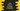# Java program to check if a number is Kaprekar number or not## Java program to check if a number is Kaprekar number or not:

In this post, we will learn how to check if a number is a Kaprekar number or not in Java. We will write one Java program that will take a number as input from the user and print if it is a Kaprekar number or not.

### Kaprekar number:

We can use the following steps to check if a number is a Kaprekar number or not:

• Find the square value of the number.
• Divide the square value into two parts. For example, if the square of the number is 565504, we can divide it in 565 and 504 or 55 and 5504 etc.
• Find the sum of these two parts. If it is equal to the number itself, it is a Kaprekar number.

For example, 2728 is a Kaprekar number.

• The square value of 2728 is 7441984.
• Divide the square value in two parts: 744 and 1984.
• The sum of these parts is 744 + 1984, which is 2728, i.e. the number itself.

### Algorithm to follow:

We will use the below algorithm in this program:

• Take the number as an input from the user.
• Find the square value of the number and save it in a different variable.
• Find the total number of digits in the square value.
• By using a loop, check the sum of all possible parts of the number.
• If the sum is equal to the number itself for any two part, it is a Kaprekar number.

Let’s write down the program.

### Java program to check Kaprekar number:

``````import java.util.Scanner;

public class Main {

public static int digitsCount(int n) {
int d = 0;

while (n > 0) {
d++;
n /= 10;
}
return d;
}

public static boolean isKaprekar(int num) {
int square = num * num;
int totalDigits = digitsCount(square);
int sum;

if (num == 1) {
return true;
}

for (int i = 1; i < totalDigits; i++) {
int power = (int) Math.pow(10, i);

if (square % power == 0) {
continue;
}

sum = (square / power) + (square % power);

if (sum == num) {
return true;
}
}
return false;
}

public static void main(String[] args) {
int no;
Scanner sc = new Scanner(System.in);

System.out.println("Enter the number: ");
no = sc.nextInt();

if (isKaprekar(no)) {
System.out.println(no + " is a Kaprekar number");
} else {
System.out.println(no + " is not a Kaprekar number");
}

}
}``````

Here,

• digitsCount method is used to find the count of digits in the number. It takes an integer as the input parameter and returns the count of digits in that integer.
• isKaprekar method is used to check if a number is Kaprekar number or not. It takes an integer value as the parameter and returns a boolean value.
• The program takes a number as an input from the user and prints a message if it is a Kaprekar number or not.

It will print output as like below:

``````Enter the number:
703
703 is a Kaprekar number

Enter the number:
333
333 is not a Kaprekar number``````

## Find all Kaprekar numbers between 1 to 10000:

You can use the same method with a loop to find all Kaprekar numbers in a range.

``````package com.company;

public class Main {

public static int digitsCount(int n) {
int d = 0;

while (n > 0) {
d++;
n /= 10;
}
return d;
}

public static boolean isKaprekar(int num) {
int square = num * num;
int totalDigits = digitsCount(square);
int sum;

if (num == 1) {
return true;
}

for (int i = 1; i < totalDigits; i++) {
int power = (int) Math.pow(10, i);

if (square % power == 0) {
continue;
}

sum = (square / power) + (square % power);

if (sum == num) {
return true;
}
}
return false;
}

public static void main(String[] args) {
for (int i = 1; i < 10000; i++) {
if (isKaprekar(i)) {
System.out.println(i + " is a Kaprekar number");
}
}
}
}``````

It will print all Kaprekar numbers between 1 to 10000:

``````1 is a Kaprekar number
9 is a Kaprekar number
45 is a Kaprekar number
55 is a Kaprekar number
99 is a Kaprekar number
297 is a Kaprekar number
703 is a Kaprekar number
999 is a Kaprekar number
2223 is a Kaprekar number
2728 is a Kaprekar number
4879 is a Kaprekar number
4950 is a Kaprekar number
5050 is a Kaprekar number
5292 is a Kaprekar number
7272 is a Kaprekar number
7777 is a Kaprekar number
9999 is a Kaprekar number``````